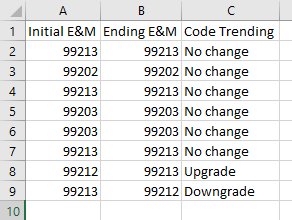# How to compare two column and update the output in column C?

Hi All,

I need to compare the 2 column “Initial E&M” and “Ending E&M” using the below conditions:

If the column Initial E&M and Ending E&M values are same value i.e 99213 and 99213
then in Column C I need to update as “No change”.

If the column Initial E&M and Ending E&M as same value i.e 99213 and 99214
then in Column C I need to update as “Upgrade”.

If the column Initial E&M and Ending E&M as same value i.e 99213 and 99212

Please do the needful do this.

Compare2Columns.xlsx (8.4 KB)

Use the following query in the Invoke Code:

``````dt.AsEnumerable.ToList.ForEach(Sub(row)
End Sub)
``````

Hope this helps,
Best Regards.

what is datatype for this??

in assign activity I need to mention.
since I need to update only C column

@Vrishchik

Use the Invoke Code activity & send the parameters this for the above case:

Edit: Output will be like-Best Regards.

If I want to update column D with same no change upgrade and downgrade means??

@Vrishchik

For the same code, just add another like to add a new column and modify the data accordingly:

``````dt.Columns.Add("Column D", GetType(String))
dt.AsEnumerable.ToList.ForEach(Sub(row)
End Sub)
``````

Best Regards.

@Vrishchik

The easiest solution would be by adding an expression. Just one assign activity does the magic.

Assign

dt.Columns(“Column C”).Expression = “IIF([Initial E&M] < [Ending E&M], ‘Upgrade’, (IIF([Initial E&M] > [Ending E&M], ‘Downgrade’,‘No Change’)))”

Can you try with these steps -

1. Use a “Read Range” activity to read the data from the source file (e.g., Excel) into a DataTable variable. Make sure to specify the range or sheet name as needed.
2. Use a “For Each Row” activity to loop through each row in the DataTable.
3. Inside the “For Each Row” loop, use the “Get Row Item” activity to access the values in the “Initial E&M” and “Ending E&M” columns for the current row. You can use the column names or column indices to retrieve the values.
4. Use an “IF” activity to compare the values using the conditions you provided. For example, you can use regular expressions to match the values. Here’s an example of a condition:

`System.Text.RegularExpressions.Regex.IsMatch(initialEMValue.ToString, “^\d{4}\$”)

`This condition checks if the “Initial E&M” value is a four-digit number using a regular expression.
5. Based on the comparison result, use an “Assign” activity to update the “Column C” value accordingly. For example, you can use the “Set Row Item” activity to update the value in the DataTable.

row(“Column C”) = “Value if true”

If the condition is false:

row(“Column C”) = “Value if false”

1. Continue looping through each row in the DataTable until all rows are processed.
2. After the loop, you can write the updated DataTable back to a file using a “Write Range” activity if needed.